Previous Page | Next Page

 Module Library

## RANDWISHART Function

generates a random sample from a Wishart distribution

RANDWISHART( N, DF, Sigma )

The inputs are as follows: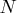is the number of desired observations sampled from the distribution.

DF
is a scalar value representing the degrees of freedom,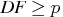.
Sigma
is a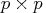symmetric positive definite matrix.

The RANDWISHART function returns an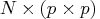matrix containingrandom draws from the Wishart distribution with DF degrees of freedom. Each row of the returned matrix represents amatrix.

The Wishart distribution is a multivariate generalization of the gamma distribution. (Note, however, that Kotz, Balakrishnan, and Johnson 2000 suggest that the term "multivariate gamma distribution" should be restricted to those distributions for which the marginal distributions are univariate gamma. This is not the case with the Wishart distribution.) A Wishart distribution is a probability distribution for nonnegative definite matrix-valued random variables. These distributions are often used to estimate covariance matrices.

If anonnegative definite matrix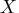follows a Wishart distribution with parameters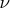degrees of freedom and asymmetric positive definite matrix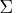, then
• the probability density function for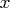is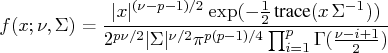• if
and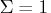, then the Wishart distribution reduces to a chi-square distribution withdegrees of freedom.
• the expected value ofis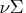.

The following example generates 1000 samples from a Wishart distribution with 7 degrees of freedom and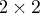matrix parameter S. Each row of the returned matrix x represents anonnegative definite matrix. (You can reshape the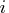th row of x with the SHAPE function.) The example then computes the sample mean and compares them with the expected value. Here are the code and the output:


call randseed(1);
N=1000;
DF = 7;
S = {1 1, 1 5};
x = RandWishart( N, DF, S );
ExpectedValue = DF * S;
SampleMean = shape( x[:,], 2, 2);
print SampleMean ExpectedValue;

SampleMean            ExpectedValue

7.0518633 14.103727         7        14
14.103727 28.207453        14        28


For further details about sampling from the Wishart distribution, see Johnson (1987, pp. 203 - 204).Previous Page | Next Page | Top of Page